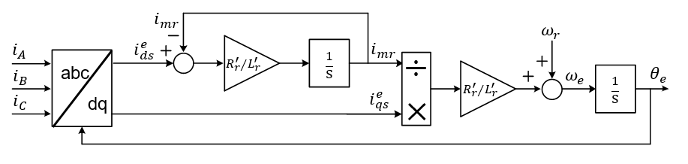# Induction Machine Flux Observer

Induction machine flux observer for field-oriented control

•Libraries:
Simscape / Electrical / Control / Observers

## Description

The Induction Machine Flux Observer block obtains the synchronous speed, ωe, and electrical angle, θe, that are required for performing rotor field-oriented control (FOC). The figure shows the equivalent circuit for the observer.### Equations

To determine the synchronous speed and electrical angle, the Induction Machine Flux Observer block uses these relationships:

`${\lambda }_{dr}^{\text{'}e}={L}_{r}^{\text{'}}{i}_{dr}^{\text{'}e}+{L}_{M}{i}_{ds}^{e}\triangleq {L}_{M}{i}_{mr},$`

`$0={R}_{r}^{\text{'}}{i}_{dr}^{\text{'}e}-\left({\omega }_{e}-{\omega }_{r}\right){\lambda }_{qr}^{\text{'}e}+\frac{d{\lambda }_{dr}^{\text{'}e}}{dt},$`

and

`${i}_{qr}^{\text{'}e}=-\frac{{L}_{M}}{{L}_{r}^{\text{'}}}{i}_{qs}^{e},$`

in these combined forms:

`${i}_{dr}^{\text{'}e}=\frac{{L}_{M}}{{L}_{r}^{\text{'}}}\left({i}_{mr}-{i}_{ds}^{e}\right)$`

`$\frac{d{i}_{mr}}{dt}=\frac{{R}_{r}^{\text{'}}}{{L}_{r}^{\text{'}}}\left({i}_{ds}^{e}-{i}_{mr}\right)$`

and

`${\omega }_{e}={\omega }_{r}+\frac{{R}_{r}^{\text{'}}}{{L}_{r}^{\text{'}}}\frac{{i}_{qs}^{e}}{{i}_{mr}}$`

where:

• ${\lambda }_{dr}^{\text{'}e}$ is the d-axis rotor flux.

• ${i}_{mr}$ is the magnetizing current.

• ${i}_{ds}^{e}$ and ${i}_{qs}^{e}$ are the d-axis and q-axis stator currents.

• ${i}_{dr}^{\text{'}e}$ and ${i}_{qr}^{\text{'}e}$ are the d-axis and q-axis rotor currents.

• ${\omega }_{e}$ is the synchronous speed.

• ${\omega }_{r}$ is the mechanical rotational speed.

• ${R}_{r}^{\text{'}}$ is the rotor resistance, referred to the stator side.

• ${L}_{r}^{\text{'}}$ is the rotor leakage inductance, referred to the stator side.

• ${L}_{M}$ the magnetizing inductance.

## Ports

### Input

expand all

Measured stator currents in the per-unit system.

Data Types: `single` | `double`

Measured rotational speed.

Data Types: `single` | `double`

### Output

expand all

Filtered d-axis and q-axis stator currents in the synchronous reference frame.

Data Types: `single` | `double`

Magnetizing rotor current.

Data Types: `single` | `double`

Rotor electrical angle.

Data Types: `single` | `double`

Rotor synchronous speed.

Data Types: `single` | `double`

## Parameters

expand all

Machine rated electrical frequency.

Rotor resistance, referred to the stator side, in the per-unit system.

Rotor leakage inductance, referred to the stator side, in the per-unit system.

Magnetizing inductance in the per-unit system.

Time constant used to low-pass filter the d-q currents.

Time, in s, between consecutive block executions. During execution, the block produces outputs and, if appropriate, updates its internal state. For more information, see What Is Sample Time? and Specify Sample Time.

If this block is inside a triggered subsystem, inherit the sample time by setting this parameter to `-1`. If this block is in a continuous variable-step model, specify the sample time explicitly using a positive scalar.

 Vas, P. Electrical Machines and Drives: A Space-vector Theory Approach. New York: Oxford University Press, 1992.